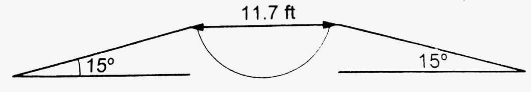Courses
Courses for Kids
Free study material
Offline Centres
MoreLast updated date: 30th Nov 2023
Total views: 277.8k
Views today: 5.77k

# A $11.7ft$ wide ditch with the approach roads at an angle of 15° with the horizontal. With what minimum speed should a motorbike be moving on the road so that it safely crosses the ditch? Assume that the length of the bike is $5{\text{ }}ft$, and it leaves the road when the front part runs out of the approach road.Verified
277.8k+ views
Hint: In order to answer the question we will assume the bike is a point object, and the ditch is $2.5{\text{ }} + {\text{ }}2.5 = 0.5$ feet wider, or $16.7$feet deep, so it leaves the road as the front portion runs out the approach road. Then, using the definition of a projectile, we will calculate the minimum speed with a projection angle of 15 degrees and a length of 16.7 feet.

Given, Width of the ditch= $11.7ft$ and Length of the bike= $5ft$. The approach road makes an angle of ${15^ \circ }\left( \alpha \right)$ with the horizontal. The biker's total horizontal width that must be protected in order to safely cross the ditch,
$R = 11.7 + 5 = 16.7\,ft$
Acceleration due to gravity,
$a = g = 9.8\,m/s \\ = \,32.2\,ft/{s^2} \\$
The horizontal range, as we know, is determined by
$R = \dfrac{{{u^2}\sin 2\alpha }}{g}$
We get, by placing respective values,
${u^2} = \dfrac{{Rg}}{{\sin 2\alpha }} \\ \Rightarrow {u^2}= \dfrac{{16.7 \times 32.2}}{{\sin {{30}^ \circ }}}$
$\therefore u \approx 32ft/\operatorname{s}$

As a result, the minimum speed at which the motorcycle can travel is $32ft/s$.

Note:The trajectory equation is the equation of projectile motion. So, if we know the x-component of an object's location, we can use the projectile motion equation to find the y-component of the position. The equation of the direction of projectile motion can be used to obtain all of the quantities involved in projectile motion, either directly or indirectly.